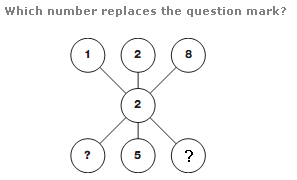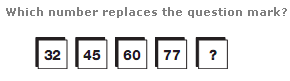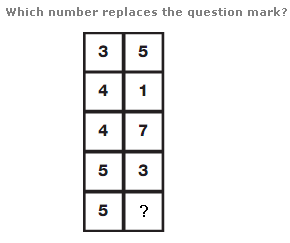# Puzzles - Number puzzles

Exercise : Number puzzles
46.:
2
Explanation
:
The diagram represents a multiplication sum : 128 x 2 = 256.

47.:
96
Explanation
:
Moving from left to right, numbers follow the sequence of square numbers, from 6 to 10, subtracting 4 each time.

48.